# Weighting data points with fitted curve in Matlab

• MATLAB
Steven Thomas
Hi all,

I'm currently in the middle of performing an experiment for the final project of my MSc, and I have a question about how I should go about weighting the data when fitting a curve to it using the MATLAB fitting tool.

Firstly, a bit of background about the problem.

I am seeing how low temperature plasmas can be used to dissociate CO2, Carbon Dioxide, into CO, Carbon Monoxide. In order to see how much CO there is after the plasma I use an FTIR and absorption spectroscopy to measure the area of part of the spectra. I am currently trying to rerun the CO calibration.

To do this, I pass known admixtures of Argon and CO, then measure the area. So I have ten values of CO percentage, 0.1% to 1.0 percent in 0.1% increments, and I have 10 corresponding areas, each having been calculated from the mean of 10 readings at each admixture, so I also have a corresponding standard deviation (σ) and standard error (SE) for each admixture.

Normally I would just fit a function with the admixture along the x-axis and the area on the y axis, weighting each point with either 1/σ2 or 1/SE2. However, as in my experiment I will be recording areas of CO curves and want a corresponding percentage out, it is better for me to fit the data the other way around, with percentage along the y-axis and area on the x axis. With the data this way round I am fitting the function f(x) = a*x2 + b*x.

Without specifying any weights to my data points, I get the fit below: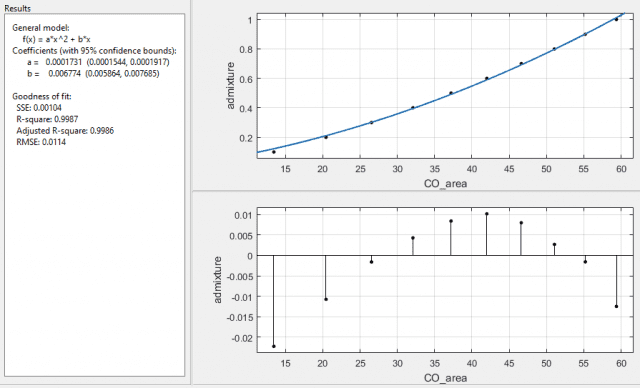with error on the coefficients as: a ±10.8%, and b ±13.4%.

With 1/σ2 as the weights I get: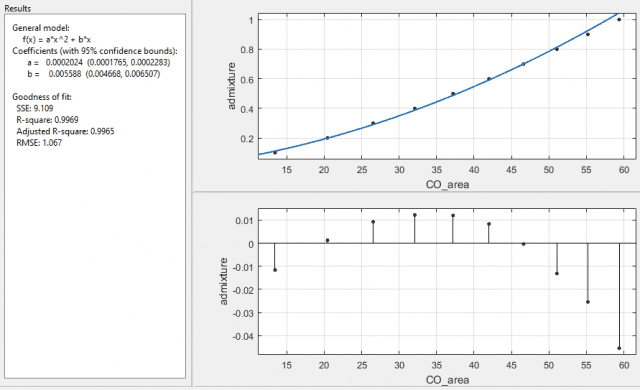and with 1/SE2 I get: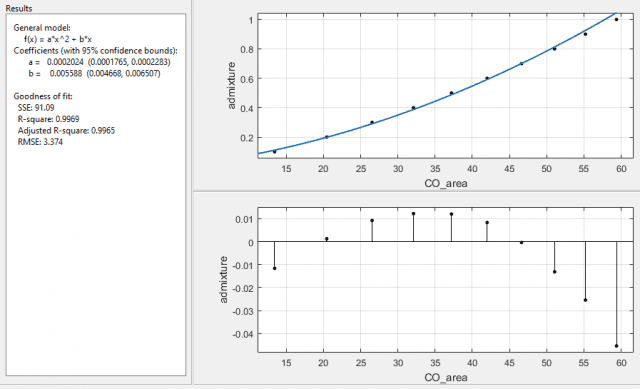both with error on the coefficients as a ±12.8%, and b ±16.5%. Interesting that the function it returns is the same, for the last two cases, with the same R-square and adjusted R-square values, but with different SSE and RMSE values. The SSE is a factor of 10 different and the RMSE is a factor of √10 different, which I assume is due to the 10 readings used for the means taken into account when using the standard error over the standard deviation. What I am a little puzzled about is why the fit is now worse when I've specified which data points it should more carefully plot to.

So finally I come to asking my question - why? Have I misinterpreted how to use the weighting function in matlab, and should I have lower numbers as the ones I want to fit more carefully to?

Or is it because I have flipped the axis round on my data, so if I was just to plot the data with error bars, they would now be horizontal rather than vertical?

I am suspecting the second to be true, as I have tried fitting the function using just σ2 and SE2, and I get the following respectively: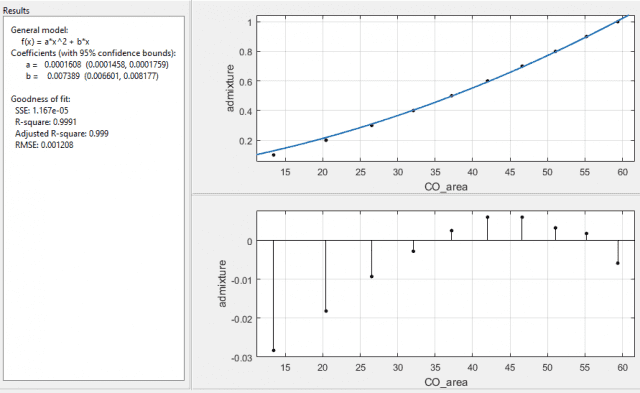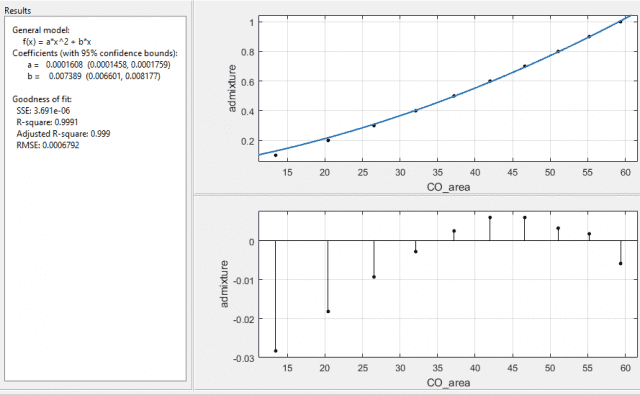again, both with the same values of the coefficients with errors a ±9.4%, and b ±10.7%. So the errors are lower, the SSE and RMSE are lower, and the R-square values are closer to 1 too.

Which way should I be doing it? Or have I got this all wrong and shouldn't be using absolute standard errors to weight with, but instead should be using percentage standard errors?

I know this is a bit of a long post, so if you've read this far I really thank you, but I've going through this for a couple of days now and would like some advice from someone better at statistics than I am.

Steve.

Homework Helper
I have not used weights in Matlab before, but I think there is a need to normalize them.
Using 1/SE seems to make the most sense, since SE is the standard error of the mean, which is what you are trying to plot.
To normalize, I would recommend something like
## w = \frac{1}{1+|\overline{SE} - SE|} ## where ##\overline{SE}## is your mean of the standard errors.
Another option would be to use
##S = \frac{|\overline{SE} - SE|}{\overline{SE}}##
And let weight be
## w = \frac{1}{1+S} ##
The effect of weights like these would be that if all your SE terms were the same, you would have all the weights be equal to 1.
Depending on the scale of your original SE, relative weights could vary a lot by just using 1/SE.
Hope this helps.

Steven Thomas
Hi @RUber

Ihave tried your method and it gives me much better error percentages on the coefficients and it also reduces the SSE and RMSE and moves the Rsquare and adjusted Rsquare closer to 1, so that's good.

I've since also tried using 1/percentage error squared, where the percentage error is the standard error / value of the data point * 100. This gives me even better errors on the coefficients, but not as good a SSE RMSE Rsquare and adjusted R square as your methods do.

I really don't know, this is why I hate stats stuff, there's no correct way to do it, but the different methods will give me completely different CO percentages and yields in my experiment.

Can I ask you about the fact I have switched my axis around so that I'm fitting a function that would now have the error bars horizontal as aposed to vertical? Do you think this means I need to change the way I would usually pick my weights for the fit?

Steven.

Homework Helper
I really don't know, this is why I hate stats stuff, there's no correct way to do it, but the different methods will give me completely different CO percentages and yields in my experiment.
Steven.
Fitting curves really is a black art. You can apply some standard methods, but when you want to apply some additional finesse, everyone will tell you it depends, so there are few authoritative standards.
By definition, the un-weighted model will minimize your un-weighted error. By changing the weights, you are allowing for more error around points with more variability and hoping for less error around points with smaller variability. I think this is a smart application of the weighted model. Choosing the weights should be done in a way that helps. It seems like your goal is to still have a pretty good fit overall, so you do not want your weights to be too far out of proportion.

Depending on your data, I would try to keep the weights between 0.5 and 1.5, so that the underweighted points aren't completely disregarded by the model.
For comparison, if one point is weighted 1.5 and another has weight of 0.5, your model would be willing to trade up to 3 units of error around the lighter point in favor of 1 less unit error on the heavier point. If you have weights ranging from 0.1 to 10, then imagine how much total error you are allowing around the "unimportant" points, maybe to get just a fraction closer to your important points.

Can I ask you about the fact I have switched my axis around so that I'm fitting a function that would now have the error bars horizontal as aposed to vertical? Do you think this means I need to change the way I would usually pick my weights for the fit?
I don't think that should matter.

Steven Thomas
That's a really clear and coherent answer thank you. I think I now know how I'll proceed. I'd like to quote it almost in my lab book as a rational for my choice, did you get that from any particular book or are you a lecturer or anything that I can put down?

Homework Helper
You can quote my username and PF as the source. I am currently an Asst. Prof of Mathematics.